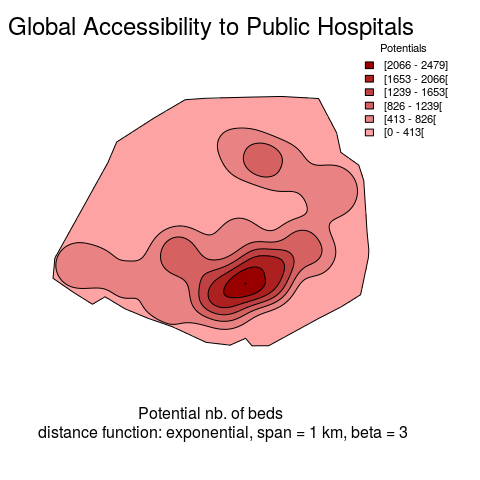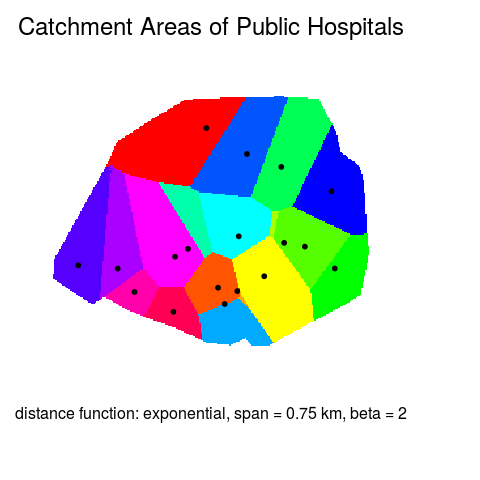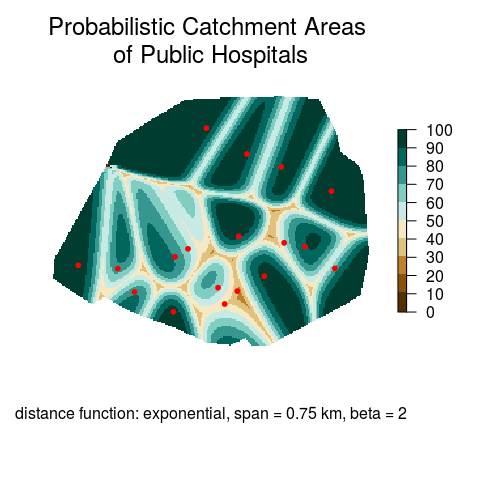# Introduction to the SpatialPosition package

## Historic and conceptual background

### Modeling spatial interactions

Modeling spatial interactions is used to understand and quantify the level of interaction between different locations. It implies carrying out the three following steps:

1. Conceptual formalization of entities (e.g. individuals, spatial units) and relationships (e.g. work, power, goods).
2. Corresponent mathematical formalization: $$T_{ij} = S_i D_j / d_{ij}^2$$ the trade flow between units i and j ($$T_{ij}$$) is proportional to the supply of unit i ($$S_i$$) and the demand of unit j ($$D_j$$) and inversely proportional to the squared distance between both units ($$d_{ij}^2$$).
3. Informatic formalization of the model: language, algorithms, etc.

The most ancient and common spatial interactions model is of the gravity form (like below). It has roots in the late 19st century (Casey, Ravenstein) and has been used in several fields (geography, economy, demography) to model a high variety of flows (commuting, trade, migrations). A brief presentation can be found in the Geography of transport systems. For a detailed one see, among many others, the book of Fotheringham and O’Kelly, Spatial Interaction Models: Formulations and Applications (Kluwer Academic Publishers, 1989). In French you can found pedagogic materials on the website of Pr. Grasland

### Place-based models: Stewart, Reilly, Huff

There are two main ways of modeling spatial interactions: the first one focuses on links between places (flows), the second one focuses on places and their influence at a distance. Following the physics metaphor, the flow may be seen as the gravitational force between two masses, the place influence as the gravitational potential. The SpatialPosition package, as its name suggests, proposes an implementation of place-based models: Stewart, Reilly and Huff models.

The Stewart model  is also known in the literature, depending on the discipline, as potential access, gravitational potential or gravitational accessibility. The concept was developped in the 1940s by physicist John Q. Stewart from an analogy to the gravity model. In his seminal work on the catchment areas of American universities, Stewart computes potentials of population. This potential is defined as a stock of population weighted by distance:

$A_i = \sum_{j=1}^n O_j f(d_{ij})$

The terms of this equation can be interpreted in a potential approach or an accessibility approach (in brackets):

• $$A_i$$ is the potential at $$i$$ (the accessibility)
• $$O_j$$ is the stock of population at $$j$$ (the number of opportunities)
• $$f(d_{ij})$$ is a negative function of the distance between $$i$$ and $$j$$, mainly of the power or the exponential form.

The computation of potentials could be considered as a spatial interpolation method such as inverse distance weighted interpolation (IDW) or kernel density estimator. These models aim to estimate unknown values of non-observed points from known values given by measure points. Cartographically speaking, they are often used to get a continuous surface from a set of discrete points. However, we argue that the Stewart model is mainly a spatial interaction modeling approach, with a possible secondary use for spatial interpolation.

The Reilly  and the Huff  models draw catchment areas. They have been widely used to study the location of economic activities and are part of the geomarketing toolbox. These models aim to quantify the attraction force on a location $$i$$ generated by an opportunity $$j$$:

$A_{ij} = O_j f(d_{ij})$

The attraction force between $$i$$ and $$j$$ ($$A_{ij}$$) is proportional to the mass of $$j$$ ($$O_j$$) and inversely proportional to the distance between both locations ($$d_{ij}$$). Let $$i$$ be the location of a consumer and $$j$$ the locations of a set of shopping malls. We compute all the attraction forces sustained by $$i$$ and we assign $$i$$ to the mall with the strongest attraction force. Computed on a regular fine grid of points $$i$$ the model produces a set of deterministic catchment areas.

The Huff model is a relative version of the Reilly model: for a given point $$i$$ and a given attraction center $$j$$, the attraction force of $$j$$ is divided by the sum of all the possible attraction centers that affect $$i$$. The result my be understood as the probability to choose $$j$$ among the set of possible destinations. Computed on a regular fine grid of points $$i$$ the model produces a raster representing probable catchment areas.

### The distance friction

Modeling spatial interactions means quantifying the distance friction or impedance. The role of the distance can be interpreted as a disincentive to access desired destinations or opportunities (e.g. jobs, shops). At the very place of the opportunity, the interaction function equals 1, meaning that the potential access is 100%. Far away from the opportunity, the interaction function tends to 0, meaning that the potential access is 0 %. The span is defined as the value where the interaction function falls to 0.5 (50%). From the individuals’ point of vue, this function may be seen as a degree of availability of a given opportunity. From the opportunity’s point of vue (a store for example), the interaction function may be seen as a decreasing catchment area: there is a maximal attraction close to the opportunity and this attraction decreases progressively through distance.

## Examples

Tha example dataset consists in three spatial objects:

• spatUnits (polygons): 20 spatial units representing the Paris’ districts (arrondissements)
• spatMask (polygons): 1 spatial unit representing the Paris’ perimeter
• spatPts (points): 18 points representing the public hospitals with their capacity (number of beds)

### Drawing global accessiblity to public hospitals

First we want to compute a global accessibility to hospitals. This computation could lead the residential choice of a highly hypocondriac individual searching for the optimal location with the best global access to hospitals. It could also lead an investment choice of the local authority searching for the optimal location to improve equity of access to health services.

The set of known points are the hospitals and the weighting variable – i.e. the mass of the points – is their capacity (Capacite). We want to create a raster of values and to clip it with the perimeter of the study area so we provide clipping mask (spatMask). The function type, the span and the exponent should reflect research hypothesis or known behavior of the individuals: e.g. in a dense urban area such as the center of Paris, an average consumer wouldn’t go farther than 500 meters to reach a small retail shop and the friction of the distance is very high (exponential form with a large beta coefficient). Here we set the span at 1000 meters, the impedance function is exponential with beta = 3.

library(SpatialPosition)
## Loading required package: raster
## Loading required package: sp
data(spatData)

# Compute potentials (accessibility)
globalAccessibility <- stewart(knownpts = spatPts, varname = "Capacite",
typefct = "exponential", span = 1000, beta = 3,
resolution = 50,

# Create a raster

# Plot the raster
par(mar = c(4,2,2,1))

plotStewart(x = rasterAccessibility, add = FALSE, nclass = 6)

# The function returns break values
breakValues <- plotStewart(x = rasterAccessibility, add = FALSE, nclass = 6)

# Create contour lines and add them to the plot
contLines <- rasterToContour(x = rasterAccessibility, levels = breakValues)

mtext("Global Accessibility to Public Hospitals", side = 3,cex = 1.5)
mtext(text = "Potential nb. of beds
distance function: exponential, span = 1 km, beta = 3",
side = 1, line = 1)### Drawing catchment areas of public hospitals

We now want to draw the catchment areas corresponing to the public hospitals. These areas could be used as a zoning tool, in order to set the residential zones with its referent hospital. As for the global accessibility, three steps are needed: computing the values (reilly), creating the raster (rasterReilly), plotting the raster (plotReilly).

row.names(spatPts)
##   "1"  "2"  "3"  "4"  "5"  "6"  "7"  "8"  "9"  "10" "11" "12" "13" "14"
##  "15" "16" "17" "18"
catchReilly <- reilly(knownpts = spatPts, varname = "Capacite",
typefct = "exponential", span = 750, beta = 2,

# Create a raster

par(mar = c(4,2,2,1))
# Plot the raster and add the points
plotReilly(x = rasterCatch)
plot(spatPts, pch = 20, add = TRUE)

mtext("Catchment Areas of Public Hospitals", side = 3,cex = 1.5)
mtext(text = "distance function: exponential, span = 0.75 km, beta = 2",
side = 1, line = 0)### Drawing probabilistic catchment areas of public hospitals

We now want to draw the probabilistic catchment areas corresponing to the public hospitals. These areas show the probability for a patient to go to one hospital or to another. It is used in geomarketing to detect the fuzzy areas where there are competing shopping malls and where the brand should focus its advertising. The same three steps are needed: computing the values (huff), creating the raster (rasterHuff) and plotting the raster (plotHuff).

###
catchHuff <- huff(knownpts = spatPts, varname = "Capacite",
typefct = "exponential", span = 750, beta = 2,

# Create a raster
side = 1, line = 0)